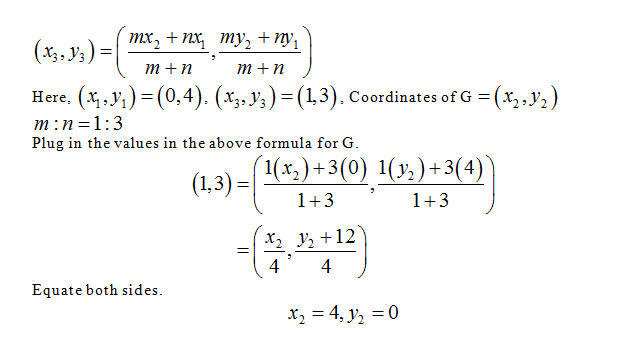# Line segment EG is partitioned by point F in the ratio 1:3. Point E is at E (0, 4), and point F is at (1, 3). What are the coordinates of point G?

Question
23 views

Line segment EG is partitioned by point F in the ratio 1:3. Point E is at E (0, 4), and point F is at (1, 3). What are the coordinates of point G?

check_circle

Step 1 If a line segment with end points (x1, y1) and (x2, y2) is partitioned in the ratio m:n then,...

### Want to see the full answer?

See Solution

#### Want to see this answer and more?

Solutions are written by subject experts who are available 24/7. Questions are typically answered within 1 hour.*

See Solution
*Response times may vary by subject and question.
Tagged in

### Math